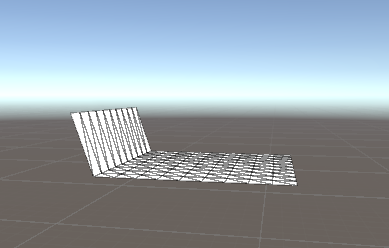# My cloth constriants dont work

im trying to create a simple cloth simulation such that it responds to forces such as gravity and inertia, im using verlet integration for this, after I got that working it was time to add the constraints but I cant get them to work, heres the code and a screenshot, details about the code are below the screenshot

``````using UnityEngine;
using System.Collections;
using System.Collections.Generic;

public class CustomCloth : MonoBehaviour
{
[SerializeField] private Vector3 constForces;
[SerializeField] private List<int> frozenVerts;

[SerializeField] private GameObject checkCube;
[SerializeField] private int Index;

private List<int> usedVerts = new List<int>();
private float reqDist;
private Vector3[] vertices, oldPos, curPos;
private Vector3 objectPrevPos;
private Mesh mesh;

void Start()
{
objectPrevPos = transform.position;
mesh = GetComponent<MeshFilter>().mesh;
vertices = mesh.vertices;

curPos = vertices;
oldPos = vertices;

reqDist = Mathf.Abs((vertices - vertices).magnitude);
}

private void FixedUpdate()
{
Simulate();
mesh.vertices = vertices;
usedVerts = new List<int>();

checkCube.transform.localPosition = vertices[Index];
}

private void Simulate()
{
for(int i = 0; i<vertices.Length; i++)
{
if (!frozenVerts.Contains(i))
{
Vector3 thisCurPos = curPos[i] + transform.position;
Vector3 thisOldPos = oldPos[i] + objectPrevPos;
Vector3 newPos = thisCurPos + (thisCurPos - thisOldPos);
newPos += constForces * Time.deltaTime;

oldPos[i] = curPos[i];
curPos[i] = newPos-transform.position;
vertices[i] = curPos[i];
}

int secondIndex = FindNearestVertexIndex(vertices[i]);
Vector3 secondVert = vertices[secondIndex];
float dist = Vector3.Distance(vertices[i], secondVert);
float error = dist - reqDist;
Vector3 changeDir = (vertices[i] - secondVert).normalized;
Vector3 changeAmount = changeDir * error;

vertices[i] -= changeAmount * 0.5f;
secondVert += changeAmount * 0.5f;
vertices[secondIndex] = secondVert;
}
objectPrevPos = transform.position;
}

int FindNearestVertexIndex(Vector3 vert)
{
int nearestVertexIndex = -1;
float nearestDistance = float.MaxValue;

for (int i = 0; i < vertices.Length; i++)
{
float distance = Vector3.Distance(vertices[i], vert);
if (distance < nearestDistance && !usedVerts.Contains(i))
{
nearestDistance = distance;
nearestVertexIndex = i;
}
}

return nearestVertexIndex;
}
}

``````Note:
Currently, I have disabled gravity
These are the results I got when I manually moved the cloth a little down
The vertices on the far left are frozen (added to a certain list of vertex indexes in my cloth script, these vertices dont respond to any forces or movements)